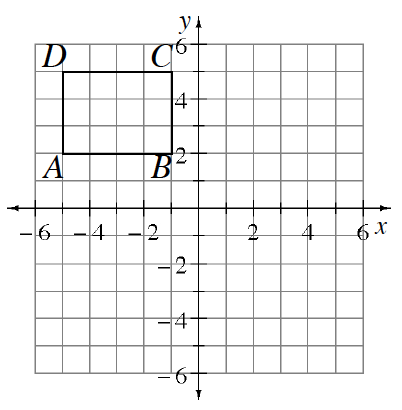Home > GB8I > Chapter cc43 > Lesson cc43.2.1 > Problem3-81

3-81.Copy rectangle ABCD at right on graph paper. 3-81 HW eTool (Desmos) Homework Help ✎.

1. Rotate rectangle ABCD 90° counterclockwise (↺) about the origin to create rectangle ABCD′. Name the coordinates of C′.

2. Reflect rectangle ABCD across the vertical line x = 1 to create rectangle ABCD″. Name the coordinates of C′′.

3. Translate rectangle ABCD so that C is moved 5 units down and 12 units right. Name the coordinates of C′′′. How is this transformation related to the line $y=−\frac{5}{12}x+1$?

4. What is the area of rectangle ABCD?

Use the eTool below to solve each part.
Click the link at right for the full version of the eTool: Int1 3-81 HW eTool.